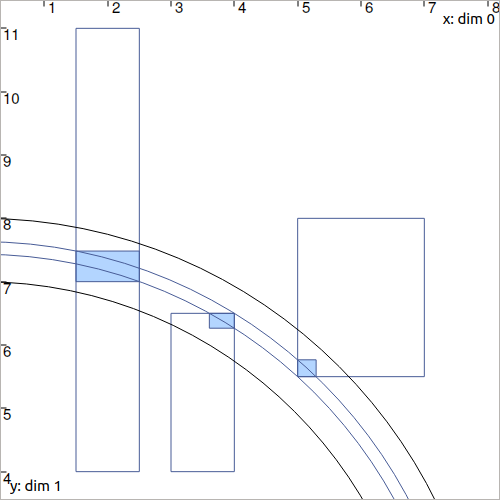# CtcDist: $$\sqrt{x^2+y^2}=d$$¶

The $$\mathcal{C}_{\textrm{dist}}$$ contractor is mainly used in robotics to express the distance constraint $$d$$ between two vectors $$\mathbf{a}$$ and $$\mathbf{b}$$. Its implementation currently stands on the $$\mathcal{C}_{\mathbf{f}}$$ contractor previously presented.

## Definition¶

Important

$\sqrt{(a_1-b_1)^2+(a_2-b_2)^2}=d \longrightarrow \mathcal{C}_{\textrm{dist}}\big([\mathbf{a}],[\mathbf{b}],[d]\big)$
ctc.dist.contract(a, b, d)

ctc::dist.contract(a, b, d);


Optimality

This contractor is optimal.

## Example¶

Suppose that we want to estimate the distance $$d$$ between a vector $$\mathbf{x}=(0,0)^\intercal$$ and several vectors $$\mathbf{b}^1$$, $$\mathbf{b}^2$$, $$\mathbf{b}^3$$.

We define domains (intervals and boxes):

• $$[d]=[7,8]$$

• $$[\mathbf{x}]=[0,0]^2$$

• $$[\mathbf{b}^1]=[1.5,2.5]\times[4,11]$$

• $$[\mathbf{b}^2]=[3,4]\times[4,6.5]$$

• $$[\mathbf{b}^3]=[5,7]\times[5.5,8]$$

d = Interval(7,8)
x = IntervalVector(2,Interval(0))
b1 = IntervalVector([[1.5,2.5],[4,11]])
b2 = IntervalVector([[3,4],[4,6.5]])
b3 = IntervalVector([[5,7],[5.5,8]])

Interval d(7.,8.);
IntervalVector x(2,0.);
IntervalVector b1{{1.5,2.5},{4,11}};
IntervalVector b2{{3,4},{4,6.5}};
IntervalVector b3{{5,7},{5.5,8}};


Several calls to $$\mathcal{C}_{\textrm{dist}}$$ will allow the contraction of both the $$[\mathbf{b}^i]$$ and $$[d]$$. Because domains are involved in several contractions, an iterative contraction loop is necessary in order to reach a consistency state (in our case, two iterations are sufficient):

ctc_dist = CtcDist()

for i in range(0,2): # iterative contractions
ctc_dist.contract(x, b1, d)
ctc_dist.contract(x, b2, d)
ctc_dist.contract(x, b3, d)

# note that we could also use directly the ctc.dist object already available

CtcDist ctc_dist;

for(int i = 0 ; i < 2 ; i++) // iterative contractions
{
ctc_dist.contract(x, b1, d);
ctc_dist.contract(x, b2, d);
ctc_dist.contract(x, b3, d);
}

// note that we could also use directly the ctc::dist object already availableFig. 16 Illustration of several contracted boxes with the above ctc_dist contractor. The blue boxes have been contracted as well as the ring.

Technical documentation